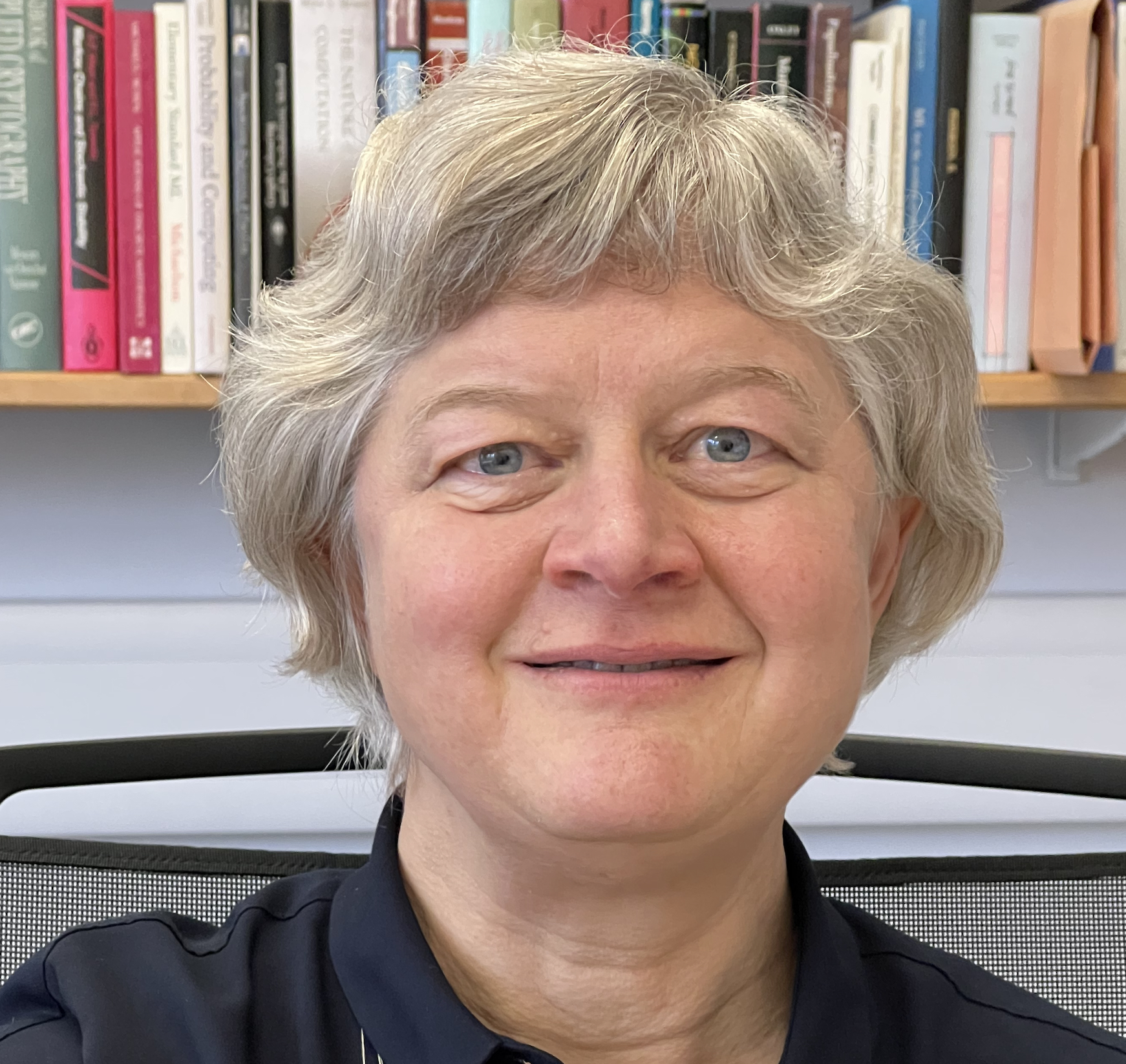• Professor Leslie Ann Goldberg

• Email (research, my teaching, or St Edmund Hall): leslie.goldberg@seh.ox.ac.uk
• Office: 256 Wolfson Building

• PA: Jayne Bullock Jayne.Bullock@cs.ox.ac.uk +44 1865 283503

• Address: Department of Computer Science, University of Oxford,
Wolfson Bldg, Parks Rd,
Oxford OX1 3QD United Kingdom

I am the Head of the Department of Computer Science (and a Professor of Computer Science) at the University of Oxford. I am also a Senior Research Fellow at St Edmund Hall. I teach the MSc and Part C course Probability and Computing.

Faculty positions at Oxford including Algorithms and Complexity Theory: See here.

Prospective PhD students:I am looking for new DPhil (PhD) students to join me in Autumn of 2022. Prospective students should feel free to get in touch if any of the projects described here sound interesting, or if you have other related ideas in algorithms or complexity theory.

## Research Interests

I am interested in foundational questions in Algorithms and Complexity Theory. A primary goal in this area is to figure out which computational problems can be solved with fast algorithms and to discover fast algorithms for solving these problems. The other primary goal is to figure out which problems provably can't be solved with fast algorithms, and to prove that no fast algorithms exist for solving these problems (usually relying on conjectures from the field of complexity theory).

I am especially interested in randomised algorithms, which are algorithms that use probabilistic methods to solve problems. Randomised algorithms arise in a huge variety of computational applications including algorithms for communication and information spread in networks, algorithms for machine learning, and algorithms for analysing computational models from statistical physics. I am particularly interested in the rigorous, mathematical analysis of these algorithms - proving results about how long the algorithms take, and how accurate they are.

My current research projects include
• Harnessing foundational methods to analyse propagation in realistic network models. This work, joint with John Lapinskas at Bristol, uses foundational methods to better analyse propagation in realistic network models. The randomised processes that we study include epidemic models, models of information spread in social networks, the Moran process (which models the spread of genetic mutations through graphs) and variants of the voter model (which models the spread of competing ideas in social networks). It also includes the study of contention-resolution processes, which apply to the spread of information. Some earlier papers in this general area include this (joint with Galanis, Goebel, Lapinskas and Richerby) and this (joint with Lapinskas and Richerby).

• Randomised algorithms for approximate counting. In terms of the underlying methods and ideas, it is only a short hop from the study of algorithms for random sampling (which is one of my main interests) to randomised algorithms for approximate counting (which is another). The rigorous study of counting complexity goes back to Valiant in the 1970s, but in the context of randomness and approximation there are many additional challenges. This is an early paper with Dyer, Greenhill, and Jerrum, which identifies a key complexity class for approximate counting, centred around a problem called #BIS, which is the problem of approximately counting the independent sets of a bipartite graph. By now there are many computational problems and classes of computational problems which are known to be equivalent to #BIS, but we still don't know whether it even has a fast approximation algorithm (with or without randomness), and this is a tantalising open problem. A lot of my current work in this area is joint with Andreas Galanis and our jointly-supervised PhD students James Stewart and Andreas Herrera Poyatos at Oxford and with our coauthors Ivona Bezakova and Daniel Stefankovic from Rochester. Here is a recent paper (with Galanis, Guo, and Yang) about algorithms for approximately counting satisfying assignments of Boolean formulas. Most of the maths that gets used in analysing algorithms for random sampling and approximate counting comes from probability or combinatorics, but sometimes other things can come up. Here is a recent paper (with Bezakova, Galanis and Stefankovic) where the analysis of approximate counting algorithms relied more on complex dynamics (which turned out to be fun!)

• Approximately counting graph homomorphisms and related structures A lot of progress in approximate counting (including randomised approximate counting) has come from understanding key graph structures such as graph homomorphisms. Often the key ideas come from the study of the structures themselves (which turn out to be complicated and fascinating!). I am currently working in this area with Marc Roth and Standa Zivny at Oxford and with our previous jointly-supervised PhD student Jacob Focke, now at CISPA. Some examples of our earlier work in the area are here and here. The last of those two links uses methods related to graph homomorphisms to obtain approximate-counting results relevant to databases.